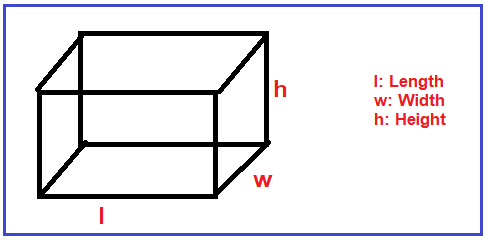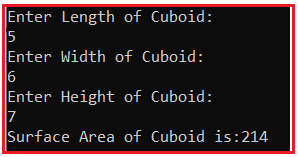# Surface Area of Cuboid in C#

## Surface Area of Cuboid Program in C#

In this article, I am going to discuss how to calculate the Surface Area of Cuboid in C# with Examples. Please read our previous article, where we discussed How to Calculate the Volume of Cube in C#. Here, in this article, first, we will discuss what is Cuboid, then we will discuss how to calculate the Surface Area of Cuboid and finally, we will see how to implement the Surface Area of Cuboid program in C#.

##### What is a Cuboid?

A cuboid is a 3-dimensional figure with a length, width, and height. A cuboid has 6 rectangular faces. The surface area of a cuboid is the sum of the area of the 6 rectangular faces that cover it.

Surface Area of Cuboid = 2 (length*width + width*height + length*height) Or

Total surface area of a cuboid (TSA) = 2lw + 2lh + 2hw = 2(lw + lh + hw)

Where l is length, w is width and h is the height. For better understanding, please have a look at the following diagram.###### Example:

Input: length=5cm, width=3cm, height=4cm
Output:
Surface area of cuboid = 2 (length*width + width*height + length*height)
Surface area of cuboid = 2(5 x 3 + 3 x 4 + 5 x 4)
= 2(15 + 12 + 20)
= 2 x 47 = 94 cm2

In this program, we used the following Data type

1. Float: Floating-point numbers are used for decimal and exponential values. It is a primitive data type. It has 6 decimal digits of precision. Example: float b = 3.4
2. Double: Double is also a data type that is used to represent the floating-point numbers. It has 15 decimal digits of precision. Example: double y = 2345.234
##### Logic to Calculate the Surface Area of Cuboid in C#

To calculate the Surface Area of Cuboid, we need to follow the below steps

1. First, we will define variables, length, width, height, surfacearea and assign the value in it.
2. After that, we will calculate the surface area of cuboids by formula. Surface area of cuboids = 2 (length*width + width*height + length*height)
3. Then calculated value store in surfacearea variable.
##### Example: Surface Area of Cuboid Program in C#.

The following C# Program will calculate the Surface Area of Cuboid.

```using System;
class AreaOfCuboid
{
static void Main()
{
float length, width, height;
length = 5;
width = 3;
height = 4;
float surfacearea = 2 * (length * width + width * height + height * length);
Console.WriteLine("Surface Area of Cuboid is:" + surfacearea);
}
}
```

Output: Surface Area of Cuboid is:94

##### Example: Taking input from the user

In the following C# program, we will take the Cuboid length, width, and height from the user and then calculate the Surface Area and print on the console.

```using System;
class AreaOfCuboid
{
static void Main()
{
Console.WriteLine("Enter Length of Cuboid:" );
Console.WriteLine("Enter Width of Cuboid:");
Console.WriteLine("Enter Height of Cuboid:");
double SurfaceArea = 2 * (Length * Width + Width * Height + Height * Length);
Console.WriteLine("Surface Area of Cuboid is:" + SurfaceArea);
}
}
```
###### Output:##### C# Code (Using function)

In the below example, we created one method to calculate the surface area of Cuboid.

```using System;
class AreaOfCuboid
{
static void Main()
{
Console.WriteLine("Enter Length of Cuboid:");
Console.WriteLine("Enter Width of Cuboid:");
Console.WriteLine("Enter Height of Cuboid:");

double SurfaceArea = CalculateSurfaceArea(Length, Width, Height);

Console.WriteLine("Surface Area of Cuboid is:" + SurfaceArea);